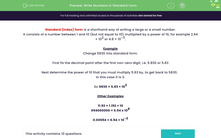# Write Numbers in Standard Form

In this worksheet, students write numbers in standard index form.Key stage:  KS 3

Curriculum topic:   Number

Curriculum subtopic:   Interpret/Compare Standard Form Numbers

Difficulty level:#### Worksheet Overview

Standard (index) form is a shorthand way of writing a large or a small number.

It consists of a number between 1 and 10 (but not equal to 10) multiplied by a power of 10, for example 2.94 × 102 or 4.8 × 10-3.

Example

Change 5830 into standard form.

First fix the decimal point after the first non-zero digit, i.e. 5.830 or 5.83

Next determine the power of 10 that you must multiply 5.83 by, to get back to 5830.

In this case it is 3.

So 5830 = 5.83 × 103

Other Examples

11.92 = 1.192 × 10

694000000 = 6.94 x 108
0.00694 = 6.94 × 10-3

### What is EdPlace?

We're your National Curriculum aligned online education content provider helping each child succeed in English, maths and science from year 1 to GCSE. With an EdPlace account you’ll be able to track and measure progress, helping each child achieve their best. We build confidence and attainment by personalising each child’s learning at a level that suits them.

Get started